Anúncio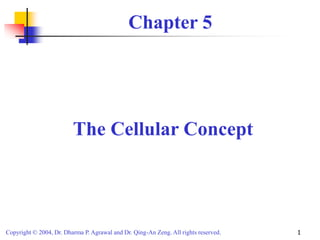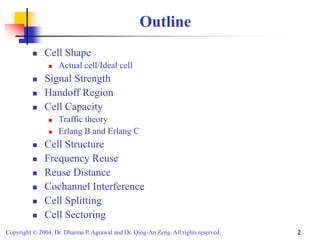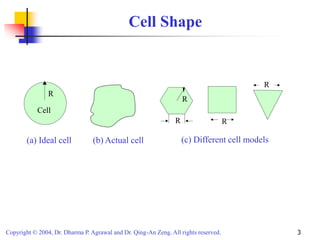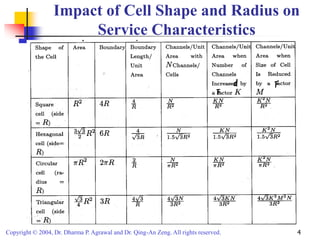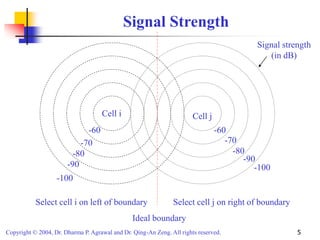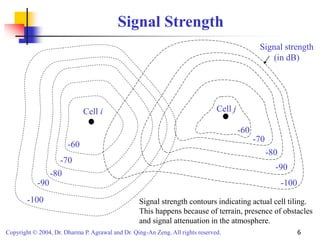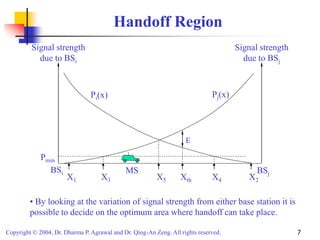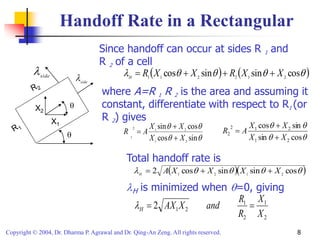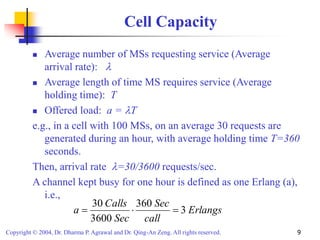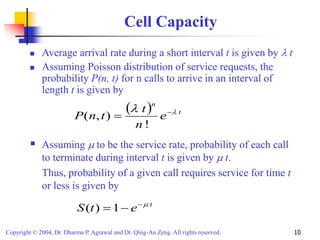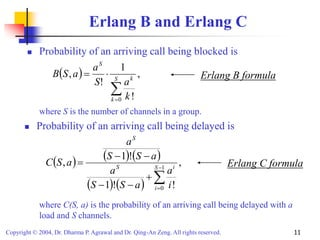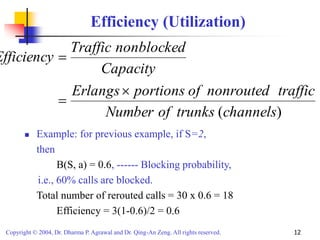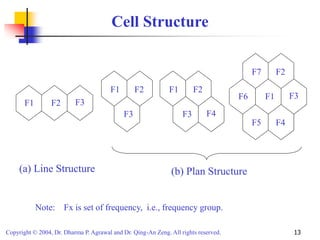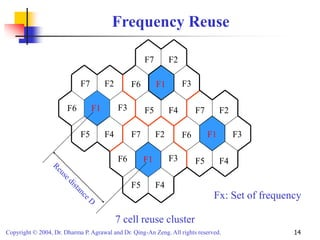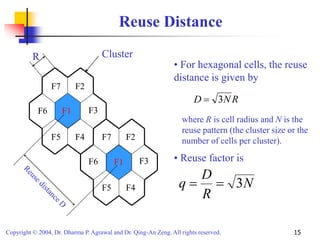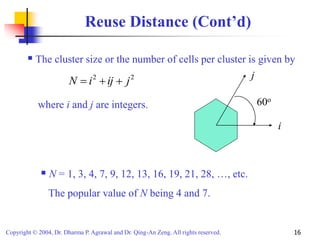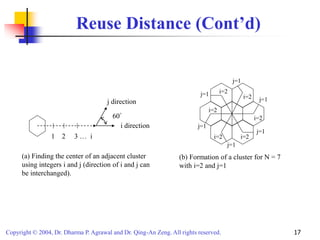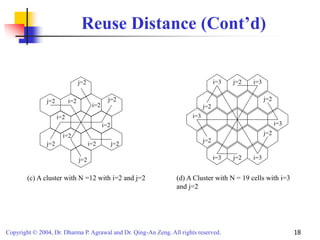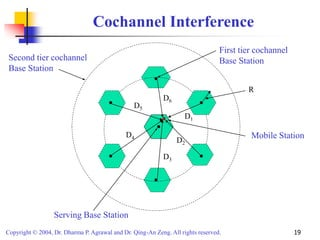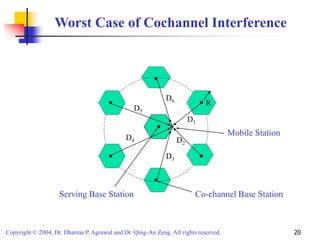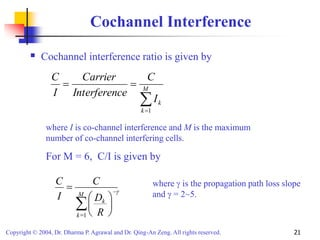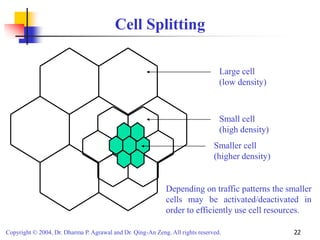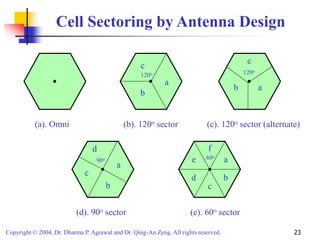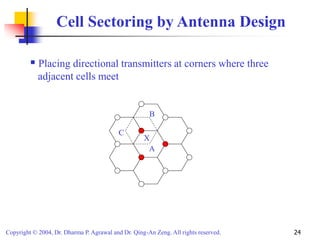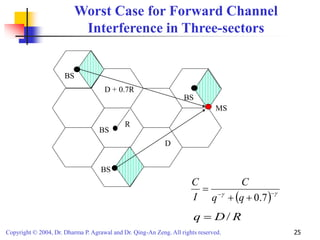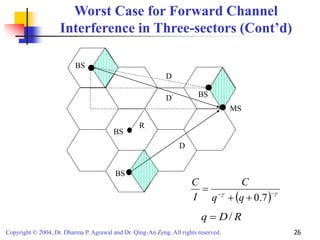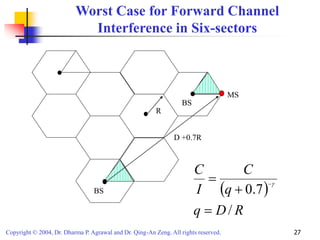1 de 27
Anúncio

### cellular 2.ppt

2. Copyright © 2004, Dr. Dharma P. Agrawal and Dr. Qing-An Zeng. All rights reserved. 2 Outline  Cell Shape  Actual cell/Ideal cell  Signal Strength  Handoff Region  Cell Capacity  Traffic theory  Erlang B and Erlang C  Cell Structure  Frequency Reuse  Reuse Distance  Cochannel Interference  Cell Splitting  Cell Sectoring
3. Copyright © 2004, Dr. Dharma P. Agrawal and Dr. Qing-An Zeng. All rights reserved. 3 Cell Shape Cell R (a) Ideal cell (b) Actual cell R R R R (c) Different cell models
5. Copyright © 2004, Dr. Dharma P. Agrawal and Dr. Qing-An Zeng. All rights reserved. 5 Signal Strength Select cell i on left of boundary Select cell j on right of boundary Ideal boundary Cell i Cell j -60 -70 -80 -90 -100 -60 -70 -80 -90 -100 Signal strength (in dB)
6. Copyright © 2004, Dr. Dharma P. Agrawal and Dr. Qing-An Zeng. All rights reserved. 6 Signal Strength Signal strength contours indicating actual cell tiling. This happens because of terrain, presence of obstacles and signal attenuation in the atmosphere. -100 -90 -80 -70 -60 -60 -70 -80 -90 -100 Signal strength (in dB) Cell i Cell j
7. Copyright © 2004, Dr. Dharma P. Agrawal and Dr. Qing-An Zeng. All rights reserved. 7 Handoff Region BSi Signal strength due to BSj E X1 Signal strength due to BSi BSj X3 X4 X2 X5 Xth MS Pmin Pi(x) Pj(x) • By looking at the variation of signal strength from either base station it is possible to decide on the optimum area where handoff can take place.
8. Copyright © 2004, Dr. Dharma P. Agrawal and Dr. Qing-An Zeng. All rights reserved. 8 Handoff Rate in a Rectangular          cos sin sin cos 2 1 2 2 1 1 X X R X X R H         sin cos cos sin 2 1 2 1 2 1 X X X X A R        cos sin sin cos 2 1 2 1 2 2 X X X X A R     X2 X1 Since handoff can occur at sides R 1 and R 2 of a cell where A=R 1 R 2 is the area and assuming it constant, differentiate with respect to R1 (or R 2) gives Total handoff rate is         cos sin sin cos 2 2 1 2 1 X X X X A H    H is minimized when =0, giving 2 1 2 1 2 1 2 X X R R and X AX H    side   side 
9. Copyright © 2004, Dr. Dharma P. Agrawal and Dr. Qing-An Zeng. All rights reserved. 9 Cell Capacity  Average number of MSs requesting service (Average arrival rate):   Average length of time MS requires service (Average holding time): T  Offered load: a = T e.g., in a cell with 100 MSs, on an average 30 requests are generated during an hour, with average holding time T=360 seconds. Then, arrival rate =30/3600 requests/sec. A channel kept busy for one hour is defined as one Erlang (a), i.e., Erlangs call Sec Sec Calls a 3 360 3600 30   
10. Copyright © 2004, Dr. Dharma P. Agrawal and Dr. Qing-An Zeng. All rights reserved. 10 Cell Capacity  Average arrival rate during a short interval t is given by  t  Assuming Poisson distribution of service requests, the probability P(n, t) for n calls to arrive in an interval of length t is given by   t n e n t t n P     ! ) , (  Assuming  to be the service rate, probability of each call to terminate during interval t is given by  t. Thus, probability of a given call requires service for time t or less is given by t e t S    1 ) (
11. Copyright © 2004, Dr. Dharma P. Agrawal and Dr. Qing-An Zeng. All rights reserved. 11 Erlang B and Erlang C  Probability of an arriving call being blocked is   , ! 1 ! , 0     S k k S k a S a a S B where S is the number of channels in a group. Erlang B formula           , ! ! 1 ! 1 , 1 0          S i i S S i a a S S a a S S a a S C Erlang C formula where C(S, a) is the probability of an arriving call being delayed with a load and S channels.  Probability of an arriving call being delayed is
12. Copyright © 2004, Dr. Dharma P. Agrawal and Dr. Qing-An Zeng. All rights reserved. 12 Efficiency (Utilization)  Example: for previous example, if S=2, then B(S, a) = 0.6, ------ Blocking probability, i.e., 60% calls are blocked. Total number of rerouted calls = 30 x 0.6 = 18 Efficiency = 3(1-0.6)/2 = 0.6 ) (channels trunks of Number traffic nonrouted of portions Erlangs Capacity nonblocked Traffic Efficiency   
13. Copyright © 2004, Dr. Dharma P. Agrawal and Dr. Qing-An Zeng. All rights reserved. 13 Cell Structure F2 F3 F1 F3 F2 F1 F3 F2 F4 F1 F1 F2 F3 F4 F5 F6 F7 (a) Line Structure (b) Plan Structure Note: Fx is set of frequency, i.e., frequency group.
14. Copyright © 2004, Dr. Dharma P. Agrawal and Dr. Qing-An Zeng. All rights reserved. 14 Frequency Reuse F1 F2 F3 F4 F5 F6 F7 F1 F2 F3 F4 F5 F6 F7 F1 F2 F3 F4 F5 F6 F7 F1 F2 F3 F4 F5 F6 F7 F1 F1 F1 F1 Fx: Set of frequency 7 cell reuse cluster
15. Copyright © 2004, Dr. Dharma P. Agrawal and Dr. Qing-An Zeng. All rights reserved. 15 Reuse Distance F1 F2 F3 F4 F5 F6 F7 F1 F2 F3 F4 F5 F6 F7 F1 F1 • For hexagonal cells, the reuse distance is given by R N D 3  R where R is cell radius and N is the reuse pattern (the cluster size or the number of cells per cluster). N R D q 3   • Reuse factor is Cluster
16. Copyright © 2004, Dr. Dharma P. Agrawal and Dr. Qing-An Zeng. All rights reserved. 16 Reuse Distance (Cont’d)  The cluster size or the number of cells per cluster is given by 2 2 j ij i N    where i and j are integers.  N = 1, 3, 4, 7, 9, 12, 13, 16, 19, 21, 28, …, etc. The popular value of N being 4 and 7. i j 60o
17. Copyright © 2004, Dr. Dharma P. Agrawal and Dr. Qing-An Zeng. All rights reserved. 17 Reuse Distance (Cont’d) (b) Formation of a cluster for N = 7 with i=2 and j=1 60° 1 2 3 … i j direction i direction (a) Finding the center of an adjacent cluster using integers i and j (direction of i and j can be interchanged). i=2 i=2 j=1 j=1 j=1 j=1 j=1 j=1 i=2 i=2 i=2 i=2
18. Copyright © 2004, Dr. Dharma P. Agrawal and Dr. Qing-An Zeng. All rights reserved. 18 Reuse Distance (Cont’d) (c) A cluster with N =12 with i=2 and j=2 i=3 j=2 i=3 j=2 i=3 j=2 i=3 j=2 i=3 j=2 i=3 j=2 (d) A Cluster with N = 19 cells with i=3 and j=2 j=2 j=2 j=2 j=2 j=2 j=2 i=2 i=2 i=2 i=2 i=2 i=2
19. Copyright © 2004, Dr. Dharma P. Agrawal and Dr. Qing-An Zeng. All rights reserved. 19 Cochannel Interference Mobile Station Serving Base Station First tier cochannel Base Station Second tier cochannel Base Station R D1 D2 D3 D4 D5 D6
20. Copyright © 2004, Dr. Dharma P. Agrawal and Dr. Qing-An Zeng. All rights reserved. 20 Worst Case of Cochannel Interference Mobile Station Serving Base Station Co-channel Base Station R D1 D2 D3 D4 D5 D6
21. Copyright © 2004, Dr. Dharma P. Agrawal and Dr. Qing-An Zeng. All rights reserved. 21 Cochannel Interference  Cochannel interference ratio is given by     M k k I C ce Interferen Carrier I C 1 where I is co-channel interference and M is the maximum number of co-channel interfering cells. For M = 6, C/I is given by          M k k R D C I C 1 g where g is the propagation path loss slope and g = 2~5.
22. Copyright © 2004, Dr. Dharma P. Agrawal and Dr. Qing-An Zeng. All rights reserved. 22 Cell Splitting Large cell (low density) Small cell (high density) Smaller cell (higher density) Depending on traffic patterns the smaller cells may be activated/deactivated in order to efficiently use cell resources.
23. Copyright © 2004, Dr. Dharma P. Agrawal and Dr. Qing-An Zeng. All rights reserved. 23 Cell Sectoring by Antenna Design 60o 120o (a). Omni (b). 120o sector (e). 60o sector 120o (c). 120o sector (alternate) a b c a b c (d). 90o sector 90o a b c d a b c d e f
24. Copyright © 2004, Dr. Dharma P. Agrawal and Dr. Qing-An Zeng. All rights reserved. 24 Cell Sectoring by Antenna Design  Placing directional transmitters at corners where three adjacent cells meet A C B X
25. Copyright © 2004, Dr. Dharma P. Agrawal and Dr. Qing-An Zeng. All rights reserved. 25 Worst Case for Forward Channel Interference in Three-sectors BS MS R D + 0.7R D BS BS BS   g g      7 . 0 q q C I C R D q / 
26. Copyright © 2004, Dr. Dharma P. Agrawal and Dr. Qing-An Zeng. All rights reserved. 26 Worst Case for Forward Channel Interference in Three-sectors (Cont’d)   g g      7 . 0 q q C I C BS MS R D’ D BS BS BS D R D q / 
27. Copyright © 2004, Dr. Dharma P. Agrawal and Dr. Qing-An Zeng. All rights reserved. 27 Worst Case for Forward Channel Interference in Six-sectors D +0.7R MS BS BS R   R D q q C I C / 7 . 0    g
Anúncio